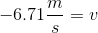# SAT II Physics : Conservation of Energy

## Example Questions

### Example Question #11 : Calculating Kinetic Energy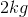book falls off the top of a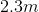bookshelf. What is its velocity right before it hits the ground?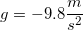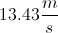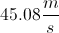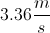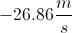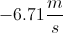Explanation:

The relationship between velocity and energy is: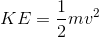We know the mass, but we need to find the total kinetic energy.

Remember the law of conservation of energy: the total energy at the beginning equals the total energy at the end. In this case, we have only potential energy at the beginning and only kinetic energy at the end. (The initial velocity is zero, and the final height is zero).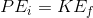If we can find the potential energy, we can find the kinetic energy. The formula for potential energy is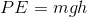.

Using our given values for the mass, height, and gravity, we can solve using multiplication. Note that the height becomes negative because the book is traveling in the downward direction.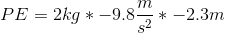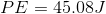The kinetic energy will also equal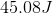, due to conservation of energy.

Using this value and our given mass, we can calculate the velocity from our original kinetic energy equation.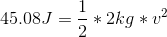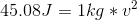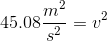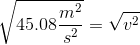Since we are taking the square root, our answer can be either negative or positive. The final velocity of the book will be in the downward direction; thus, our final velocity should be negative.